SYLLABUS  Previous: 4.4.1 Naive implementation using  Up: 4.4 Methods for European  Next: 4.5 Methods for European

4.4.2 Improved scheme using log-normal variables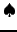[ SLIDE scheme - BC/TC - interpolation - code - run || same VIDEO as previous section: modem - LAN - DSL)]

Instead of solving Black-Scholes (3.4#eq.4) with finite differences in the financial variables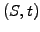, apply the transformations (4.3.1#eq.1, 4.3.1#eq.4) and solve the normalized equation (4.3.1#eq.5) with finite differences in log-normal variables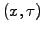.
Apart from a numerical accuracy in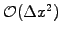, which gets independent of the underlying value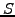, the transformation has the additional advantage of evolving the numerical solution everywhere at the same rate, so that the time step is everywhere limited by the condition for the normalized diffusion equation: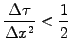(4.4.2#eq.1)

The finite difference solution is extremely simple to calculate using a difference forward from the time level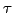(4.4.1#eq.1 since a scheme backward in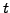runs forward in) and a second derivative centered on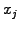(4.4.1#eq.4):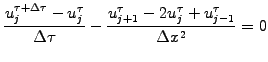(4.4.2#eq.2)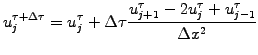(4.4.2#eq.3)

The only difficulty (which results in quite a bit of coding) is that the regular spacing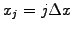assumed for the finite differencing becomes exponential in financial variable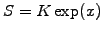. This is not a problem for the put and call options (exercise 4.08), since the terminal and boundary conditions are known explicitly in log-normal variables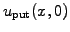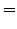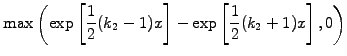(4.4.2#eq.4)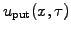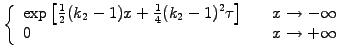(4.4.2#eq.5)

The transformation is however an additional source of imprecision when a general payoff from a binary option has first to be transformed from regularly spaced financial variables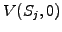to log-normal variables on an inhomogeneous grid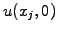, before it is interpolated onto a regular gridusing a linear approximation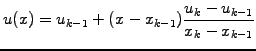(4.4.2#eq.6)

The evolution can be calculated keeping only financial variables, but an interpolation is again needed when the solution is finally plotted in the financial world. This finally yields a rather clumsy scheme that has been implemented in the VMARKET class FDSolution.java
double x0, x1, f0, f1, xk;                         //Change variables
double tau = 0.5*sigmaSq*time;                     // f(x,t) ->
double dtau= 0.5*sigmaSq*timeStep;                 //    fm(xk,tau)
double xk0 = Math.log(x/strike);                // lognormal mesh
double xkn = Math.log(x[n]/strike);
double dxk =(xkn-xk0)/(n-1);
double k1  = 2*rate/sigmaSq;
double k2  = 2*(rate-divid)/sigmaSq;
double k2m1= k2-1.;
double k2p1= k2+1.;
int    j,k;
//=== Interpolate only once from (x,t) to lognormal variables (xk,tau)
if (time<=timeStep) {
if(isPut) { //Initialize fm[] directly as put-option
for (k=1; k<=n; k++) {
xk=xk0+(k-1)*dxk;
fm[k]=Math.max(0., Math.exp(0.5*k2m1*xk) -
Math.exp(0.5*k2p1*xk)  );
}
} else if (isCall) { //Left as an exercise
} else { //Interpolate fm[] from IC in f[]
j=1; ; x0=xk0;
f0=f/strike*Math.exp(0.5*k2m1*xk0);
x1=x0; f1=f0;
for (k=1; k<n; k++) { //Loop over lognormal mesh index
xk=xk0+(k-1)*dxk;   // given xk, find x0,x1 | x[j] < xk < x[j+1]
while (xk>=x1) { j++; x0=x1; f0=f1; x1=Math.log(x[j]/strike); }
f1=f[j]/strike*Math.exp(0.5*k2m1*x1);
fm[k]= f0 + (xk-x0)*(f1-f0)/(x1-x0);
}
fm[n]= fm[n-1] + dxk*(fm[n-1]-fm[n-2]);
}
} else { //Retrieve fm[] from previous time step
}
//===== Solve diffusion equation with an explicit 2 levels scheme
double D = dtau/(dxk*dxk);
for (j=2; j<n; j++)
f[j]= fm[j] + D*(fm[j+1]-2.*fm[j]+fm[j-1]);
if (isPut) {
f= Math.exp(0.5*k2m1*xk0+0.25*k2m1*k2m1*tau);
f[n]= f[n-1];
fp=strike*Math.exp(-rate*time);
//    } else if (isCall) { //Left as an exercise
} else {
f= f;
f[n]= f[n-1];
fp=fp;
}
//===== Interpolate rest from lognormal to financial mesh variables
k=1; x0=x; x1=x0; f0=fp;
xk=xk0; f1=f*strike*Math.exp(-0.5*k2m1*xk-(0.25*k2m1*k2m1+k1)*tau);
for (j=1; j<n; j++) { //Loop over financial mesh index
while (x[j]>=x1){
k++;x0=x1;f0=f1;xk=xk0+(k-1)*dxk;x1=strike*Math.exp(xk);}
f1=f[k]*strike*Math.exp(-0.5*k2m1*xk-(0.25*k2m1*k2m1+k1)*tau);
fp[j]= f0 +(x[j]-x0)/(x1-x0)*(f1-f0);              //Lin interpol in x
}
if (isPut) {
fp[n]=f[n]*strike*Math.exp(-0.5*k2m1*xkn-(0.25*k2m1*k2m1+k1)*tau);
//    } else if (isCall) { //Left as an exercise
} else {
fp=fp;
fp[n]=fp[n-1];
}

 Virtual market experiments: log-normal FD scheme Press Step 1 after initializing different payoffs to visualize the initial error induced by the interpolation to log-normal variables and back. Reduce the interpolation error by increasing MeshPoints; note that you then have to reduce TimeStep in agreement with (4.4.2#eq.1). Switch from European logn to European and compare the results from the naive scheme with the present one using log-normal variables.

SYLLABUS  Previous: 4.4.1 Naive implementation using  Up: 4.4 Methods for European  Next: 4.5 Methods for European